﻿ Vibration Analysis of IPM MotorExamples | Product | Murata Software Co., Ltd.Example2Vibration Analysis of IPM Motor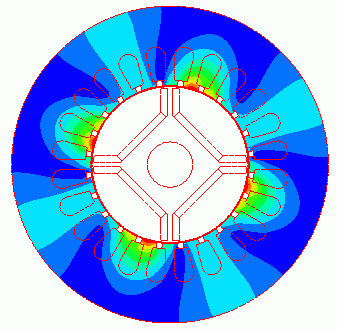General

• The vibration of a stator by the electromagnetic force of the IPM motor is analyzed.

• Displacement, stress, torque, magnetic flux density, and iron loss are solved.

• The analysis is coupled with the external circuit.

• The homogenizing method is applied to simulate the layer structure of the electromagnetic plates of steel for the core.

• Unless specified in the list below, the default conditions are applied.

Analysis Condition

 Item Setting Analysis Space 2D Thickness in Depth Direction 60[mm] Unit mm Solver Magnetic Analysis [Luvens] Analysis Type Transient Analysis Analysis Options Select External Circuit Coupling. Select Rotating machinery.   Select Planar Strain. [Reference type of electromagnetic force of the magnetic transient analysis results] Select Value at each step.

The Rotating Machinery tab is set as follows.

 Tab Setting Item Setting Rotating Machinery Rotational Movement Select Constant Velocity The Number of Rotations: 1800[r/min] Rotor’s Initial Rotation Position: 0[deg] Number of Sliding Mesh Divisions Circumferential Division Angle: 1.0[deg] Rotational Quantity per Step: 1[mesh] Number of Sliding Mesh Layers: 3

External circuit is as follows.

3-phase AC with 60Hz and 5A is applied.

The U phase is calculated with a function to [search for power phase at the maximum torque].

As [Conversion of external circuit I/O values] is ON, the resistance value of the FEM coil is set to the phase resistance which is equivalent to a full model instead of a quarter model.

See [External Circuit Conversion] and [Example of External Circuit Conversion Setup] for the details of conversion.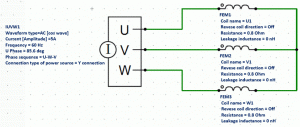Set the Mesh Tab as follows.

 Tab Setting Item Setting Mesh Meshing Setup Set the general mesh size automatically: Deselect General Mesh size: 1[mm] Automatic Ambient Air Creation Select Create ambient air automatically Ambient Air Scale: 1.2

The Transient Analysis tab is set up as follows.

The number of steps is 90, circumferential division angle is 1.0[deg], and rotational quantity per step is 1[mesh]. The rotation up to 90 degrees (=90*1.0*1) of the mechanical angle is analyzed.

It can be converted to the electric angle of 180[deg] (mechanical angle x number of poles=90*4/2). Half period of the electric angle is analyzed.

• As the input power is specified by current, the coil current is in the steady state from the beginning. The torque is also in the steady state.

• To calculate the iron loss, it is necessary to set the timesteps for one period of electric angle in the steady state, and to define the iron loss characteristic for the material property.

Tab

Setting Item

Setting

Transient Analysis

Timestep

Automatic

Table

 Number Calculation steps Output interval 1 90 1

Model

A rotor core and magnets are placed in the center. A stator and coils are placed around them.

The motor has 4 poles and 24 slots.

This is a 2D model analysis.

The stress analysis is performed on the stator domain only. The rotational displacement around the stator is fixed by the boundary condition [Fix].

• Generally, if only magnetic analysis is executed, a period-symmetric model is used which utilizes the symmetry of the model as in Example 1 of magnetic transient analysis.
In this example, however, a full model is used because a periodic boundary is not supported in the stress analysis.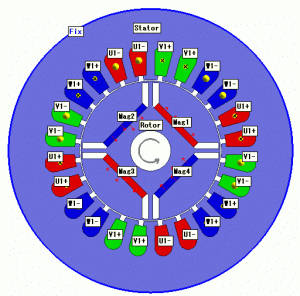Body Attributes and Materials

 Body Number/Type Body Attribute Name Material Name 14/Sheet Mag1 NMX-S43SH_Hitachi Metal* 15/Sheet Mag2 NMX-S43SH_Hitachi Metal* 63/Sheet Mag3 NMX-S43SH_Hitachi Metal* 62/Sheet Mag4 NMX-S43SH_Hitachi Metal* 66/Sheet Rotor 35JN270_JFE Steel* 65/Sheet Stator 35JN270_JFE Steel* 37/Sheet U1+ 008_Cu * 38/Sheet U1+ 008_Cu * 39/Sheet W1- 008_Cu * 40/Sheet W1- 008_Cu * 41/Sheet V1+ 008_Cu * 42/Sheet V1+ 008_Cu * 43/Sheet U1- 008_Cu * 44/Sheet U1- 008_Cu * 45/Sheet W1+ 008_Cu * 46/Sheet W1+ 008_Cu * 47/Sheet V1- 008_Cu * 48/Sheet V1- 008_Cu *

* Available from the material DB

The body attribute is set up as follows.

For the core, the homogenizing method is selected to simulate the layered steel plates.

 Body Attribute Name Tab Setting Mag1 Direction Vector: X=1, Y=0, Z=1 Current Click Yes for induced current setting. Stator/Rotor/Air Rotor Analysis Domain Deselect Stress Analysis. Mag2 Direction Vector: X=1, Y=0, Z=-1 Current Click Yes for induced current setting. Stator/Rotor/Air Rotor Analysis Domain Deselect Stress Analysis. Mag3 Direction Vector: X=-1, Y=0, Z=-1 Current Click Yes for induced current setting. Stator/Rotor/Air Rotor Analysis Domain Deselect Stress Analysis. Mag4 Direction Vector: X=-1, Y=0, Z=1 Current Click Yes for induced current setting. Stator/Rotor/Air Rotor Analysis Domain Deselect Stress Analysis. Rotor Layer Select Take layer into account Space: 97[%] Layer direction vector: X=0, Y=1, Z=0 Stator/Rotor/Air Rotor Analysis Domain Deselect Stress Analysis. Stator Layer Select Take layer into account Space: 97[%] Layer direction vector: X=0, Y=1, Z=0 Stator/Rotor/Air Stator U1+ Current Waveform: External circuit coupling Coil name on the circuit: U1 Turns: 35[Turns] Direction: +Y direction Stator/Rotor/Air Stator V1+ Current Waveform: External circuit coupling Coil name on the circuit: V1 Turns: 35[Turns] Direction: +Y direction Stator/Rotor/Air Stator W1- Current Waveform: External circuit coupling Coil name on the circuit: W1 Turns: 35[Turns] Direction: – Y direction Stator/Rotor/Air Stator U1- Current Waveform: External circuit coupling Coil name on the circuit: U1 Turns: 35[Turns] Direction: – Y direction Stator/Rotor/Air Stator V1- Current Waveform: External circuit coupling Coil name on the circuit: V1 Turns: 35[Turns] Direction: – Y direction Stator/Rotor/Air Stator W1+ Current Waveform: External circuit coupling Coil name on the circuit: W1 Turns: 35[Turns] Direction: +Y direction Stator/Rotor/Air Stator

Boundary Condition

Set the boundary condition to fix the rotational displacement surrounding the stator.

 Boundary Condition Name/Topology Tab Boundary Condition Type Setting Fix Mechanical Rotational displacement Coordinates on the axis: 0.0, 0.0, 0.0 Rotation angle: 0[deg]

Results

Below is a result of the magnetic analysis.

The distribution of the magnetic flux density and magnetic flux lines at the rotation angle of 0 [deg] are shown below.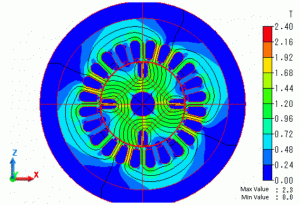The wave of torque is shown as follows. It is output to “Torque [N*m]” of the result table.

The torque is in the steady state from 0 [deg] as the current source is used.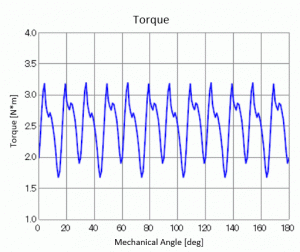Next is a result of the stress analysis.

The acceleration at the rotation angle of 10[deg] is shown below.

The vibration of the stator can be observed.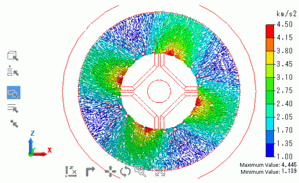﻿